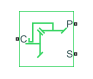# Sun-Planet Bevel

Planetary gear set of carrier and beveled planet and sun wheels with adjustable gear ratio, assembly orientation, and friction losses

•Libraries:
Simscape / Driveline / Gears / Planetary Subcomponents

## Description

The Sun-Planet Bevel gear block represents a carrier and beveled planet and sun wheels. The planet is connected to and rotates with respect to the carrier. The planet and sun gears corotate with a fixed gear ratio. You control the direction of rotation by setting the assembly orientation left or right. For model details, see Equations.

### Thermal Model

You can model the effects of heat flow and temperature change by enabling the optional thermal port. To enable the port, set Friction model to ```Temperature-dependent efficiency```.

### Equations

Ideal Gear Constraints and Gear Ratios

The Sun-Planet Bevel block imposes one kinematic and one geometric constraint on the three connected axes:

`${r}_{C}{\omega }_{C}={r}_{S}{\omega }_{S}±{r}_{P}{\omega }_{P}$`

`${r}_{C}={r}_{S}±{r}_{P}$`

where:

• rC is the radius of the carrier gear.

• ωC is the angular velocity of the carrier gear.

• rS is the radius of the sun gear.

• ωS is the angular velocity of the sun gear.

• rP is the radius of the planet gear.

• ωP is the angular velocity of the planet gear.

The planet-sun gear ratio is defined as

`${g}_{PS}=\frac{{r}_{P}}{{r}_{S}}=\frac{{N}_{P}}{{N}_{S}},$`

where:

• gPS is the planet-sun gear ratio. As ${r}_{P}>{r}_{S}$, ${g}_{PS}>1$.

• NP is the number of teeth in the planet gear.

• NS is the angular velocity of the sun gear.

In terms of this ratio, the key kinematic constraint is:

• ${\omega }_{S}={g}_{PS}{\omega }_{P}-{\omega }_{C}$ for a left-oriented bevel assembly

• ${\omega }_{S}={g}_{PS}{\omega }_{P}+{\omega }_{C}$ for a right-oriented bevel assembly

The three degrees of freedom reduce to two independent degrees of freedom. The gear pair is (1,2) = (S,P).

Warning

The planet-sun gear ratio, gPS, must be strictly greater than one.

The torque transfer is defined as

`${\tau }_{P}={\tau }_{loss}-{g}_{PS}{\tau }_{S},$`

where:

• τloss is the torque loss.

• τs is the torque for the sun gear.

• τp is the torque for the planet gear.

In the ideal case where there is no torque loss, τloss = 0. The resulting torque transfer equation is ${\tau }_{P}={g}_{PS}{\tau }_{S}$.

Nonideal Gear Constraints and Losses

In the nonideal case, τloss ≠ 0. For more information, see Model Gears with Losses.

### Variables

Use the Variables settings to set the priority and initial target values for the block variables before simulating. For more information, see Set Priority and Initial Target for Block Variables.

### Assumptions and Limitations

• Gear inertia is assumed to be negligible.

• Gears are treated as rigid components.

## Ports

### Conserving

expand all

Rotational mechanical conserving port associated with the planet gear carrier.

Rotational mechanical conserving port associated with the planet gear.

Thermal conserving port associated with heat flow. Heat flow affects the power transmission efficiency by altering the gear temperatures.

#### Dependencies

To enable this port, set Friction model to `Temperature-dependent efficiency`.

## Parameters

expand all

### Main

Ratio, gPS, of the planet gear to sun gear rotations as defined by the number of planet gear teeth divided by the number of sun gear teeth. This gear ratio must be strictly greater than 1.

Relative orientation of the sun and planet gears and their corotation direction. Left or right orientation implies that the gears corotate in the same or opposite direction, respectively.

### Meshing Losses

Friction model for the block:

• `No meshing losses - Suitable for HIL simulation` — Gear meshing is ideal.

• `Constant efficiency` — Transfer of torque between the gear wheel pairs is reduced by a constant efficiency, η, such that 0 < η ≤ 1.

• `Temperature-dependent efficiency` — Transfer of torque between the gear wheel pairs is defined by the table lookup based on the temperature.

Torque transfer efficiency, ηPS, for the planet gear to the sun gear pair meshing. This value must be greater than `0` and less than or equal to `1`.

#### Dependencies

To enable this parameter, set Friction model to `Constant efficiency`.

Vector of temperatures used to construct a 1-D temperature-efficiency lookup table. The vector elements must increase from left to right.

#### Dependencies

To enable this parameter, set Friction model to `Temperature-dependent efficiency`.

Vector of output-to-input power ratios that describe the power flow from the outer planet gear to the inner planet gear, ηPS. The block uses the values to construct a 1-D temperature-efficiency lookup table.

Each element is an efficiency that relates to a temperature in the Temperature vector. The length of the vector must be equal to the length of the Temperature vector. Each element in the vector must be in the range (0,1].

#### Dependencies

To enable this parameter, set Friction model to ```Temperature-dependent efficiency```.

Power threshold, pth, above which full efficiency is in effect. Below this value, a hyperbolic tangent function smooths the efficiency factor.

When you set Friction model to `Constant efficiency`, the block lowers the efficiency losses to zero when no power is transmitted. When you set Friction model to `Temperature-dependent efficiency`, the block smooths the efficiency factors between zero when at rest and the values provided by the temperature-efficiency lookup tables at the power thresholds.

#### Dependencies

To enable this parameter, set Friction model to `Constant efficiency` or ```Temperature-dependent efficiency```.

### Viscous Losses

Viscous friction coefficient, μS, for the sun-carrier gear motion.

### Thermal Port

To enable these settings, set Friction model to `Temperature-dependent efficiency`.

Thermal energy required to change the component temperature by a single temperature unit. The greater the thermal mass, the more resistant the component is to temperature change.

#### Dependencies

To enable this parameter, set Friction model to ```Temperature-dependent efficiency```.

expand all

## Version History

Introduced in R2011a